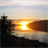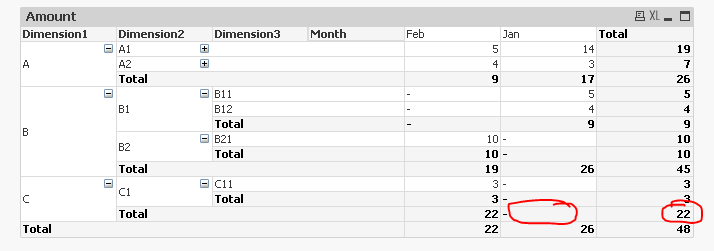# QlikView App Dev

Discussion Board for collaboration related to QlikView App Development.

Announcements
Now Live: Qlik Sense SaaS Simplified Authoring – Analytics Creation for Everyone: READ DETAILS
cancel
Showing results for
Did you mean:Contributor II

## Cumulative Dimension

Hello,

I'm facing an issue about rangesum function in a Pivot table,

I calculate a cumulative amount like this

The expression:

if((Dimensionality())=1,

rangesum( sum(  aggr(   rangesum(   above(  Sum( {\$<expr>} Amount)  ,0,rowno(TOTAL)  )  )  , Dimension 1  )  )

, sum (Amount)

I have 2 dimensions:

Dimension1 diplayed in rows and Dimension2 displayed in Columns.

This is how we want to display the pivot table:

 Dimension1 Dimension3 Dimension4 Dimension2 Z1 Z2 Total A A1 10 20 30 A A2 A21 1 3 4 A A2 A22 1 2 3 A A2 A23 2 4 6 A A2 A24 1 5 6 A A2 Total 4 14 18 A Total 14 34 48 B B1 2 3 5 B Total 16 37 53 C 17 38 55 D 18 39 57 E 19 40 59 F F1 1 2 3 G Total 20 42 62 Total 20 42 62

And for the moment I have this:

 Dimension1 Dimension3 Dimension4 Dimension2 Z1 Z2 Total A A1 10 20 30 A A2 A21 1 3 4 A A2 A22 1 2 3 A A2 A23 2 4 6 A A2 A24 1 5 6 A A2 Total 4 14 18 A Total 34 0 34 B B1 2 3 5 B Total 53 0 53 C 55 0 55 D 57 0 57 E 59 0 59 F F1 3 0 3 G Total 62 0 62 Total 62 0 62

Does anyone has any idea on how I could fix this issue?

Many thanks,

Nacera

Ce message a été modifié par : nacera abbas

1 Solution

Accepted SolutionsMVP

Try this

If(Dimensionality() = 1,

Sum(Aggr(RangeSum(Above(Sum(Amount), 0, RowNo())), Month, Dimension1)),

Sum(Amount))But because of some missing data, you will see that circle cells don't really match to what you want. To fix this, you will probably need to fix this in the script.

14 RepliesContributor II
Author

Does anyone can help on this?MVP

What is A1, A2 here? How you are you doing RangeSum on alphanumeric stuff like A1, A2? I am confusedContributor II
Author

The RangeSum is made on the Amount not the Dimension, A1 and A2 are value frome the Dimension3Contributor II
Author

I add a sample .qvw to be more clear.

As you can see below, for the first subtotal for Dimension1=A for Feb I have nothing and for Jan I have the Cumulative sum of Jan and Feb, I would like to see 9 for Feb and 17 for Jan and continue to cumulate at subtotoal for Dimension1=B Amount=A+B here as you can see 45= 26 for A and 19 for BMany thanks,

NaceraMVP

May be this

If(Dimensionality() = 1 and SecondaryDimensionality() = 0,

Sum(Aggr(RangeSum(Above(TOTAL Sum(Amount), 0, RowNo(TOTAL))), Dimension1)),

Sum(Amount))Contributor II
Author

It looks nice,

But I want the cumulative in B and C forJan and Fab as well, like below:

 Dimension1 Dimension2 Dimension3 Month Jan Feb Total A A1 14 5 19 A A2 3 4 7 A Total 17 9 26 B B1 9 - 9 B B2 - 10 10 B Total 26 19 45 C C1 - 3 3 C Total 26 22 48 Total 26 22 48

Thanks,

NaceraContributor II
Author

No one has an idea for this?MVP

Try this

If(Dimensionality() = 1,

Sum(Aggr(RangeSum(Above(Sum(Amount), 0, RowNo())), Month, Dimension1)),

Sum(Amount))But because of some missing data, you will see that circle cells don't really match to what you want. To fix this, you will probably need to fix this in the script.Contributor II
Author

It worked, many thanks stalwar1‌!!!